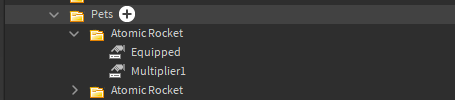# Need help fixing script

Its a System where when you have a pet or Multiple Pets equipped you get a multiplier from each pet and combine those multipliers to add more points / currency to the player when player does something where he gets Currency.

(Folder inside Player, contains all of the player’s owned pets.)Script inside ClickDetector that gives Money when clicked ( for example )

``````local ClickDetector = script.parent

function getMultiplier(Player, Multiplier1)
local Multi = 1
for i,v in pairs(Player.Pets:GetChildren()) do
if v.Equipped.Value == true then
Multi = Multi + v[Multiplier1].Value
end
end
return Multi
end
function onMouseClick(Player)
-- Here should be the rest of the script , but it has nothing to do with this topic.
-------------------------
local Multiplier1 = getMultiplier(Player)
local pointsamount = game.ServerStorage.Points.Value * Multiplier1
end

clickDetector.MouseClick:Connect(onMouseClick)
``````

I keep getting errors like:

`````` Workspace.Money.ClickDetector.Script:46: invalid argument #2 (string expected, got nil)  -  Server - Script:46

``````

You don’t need the extra `:Connect` over there.

1 Like

Oh , sorry Rookie mistake.
I am still having trouble with the script tho.

What does that argument represent? The error is with that one

Multiplier1 is supposed to be the final multiplier amount , it should get the multiplier from the “getMultiplier” function.
getMultiplier is supposed to search all of the players “pets” if they are equipped, then it should get all of the equipped ones and get their multipliers (a value called Multiplier1) values, and finally have an amount combined from all of the multiplier values.

Then what it does in the end is it multiplies the money/points your supposed to recieve from this MouseClick with the final multiplier value.
(Sorry if terrible explanation)

try replace

``````local Multiplier1 = getMultiplier(Player)
``````

To

``````local Multiplier1 = getMultiplier(Player,Multiplier1)
``````

I don’t have it anywhere.
Its not global and it doesn’t know what it means.
I don’t have another Multiplier1

Supposed to be the Multiplier value that I tried getting in the getMultiplier function

If you’re still getting the same error, where’s line 46 in the click detector script?

``````			Multi = Multi + v[Multiplier1].Value
``````

The full script is not in there, but thats just for example like some color changing on the block the clickdetector is inside of. Nothing to do with these Multipliers and points

Try replacing that line with:

``````Multi = Multi + v.Multiplier1.Value
``````

Oh god I really spend all of this time for it to be a simple soulution.